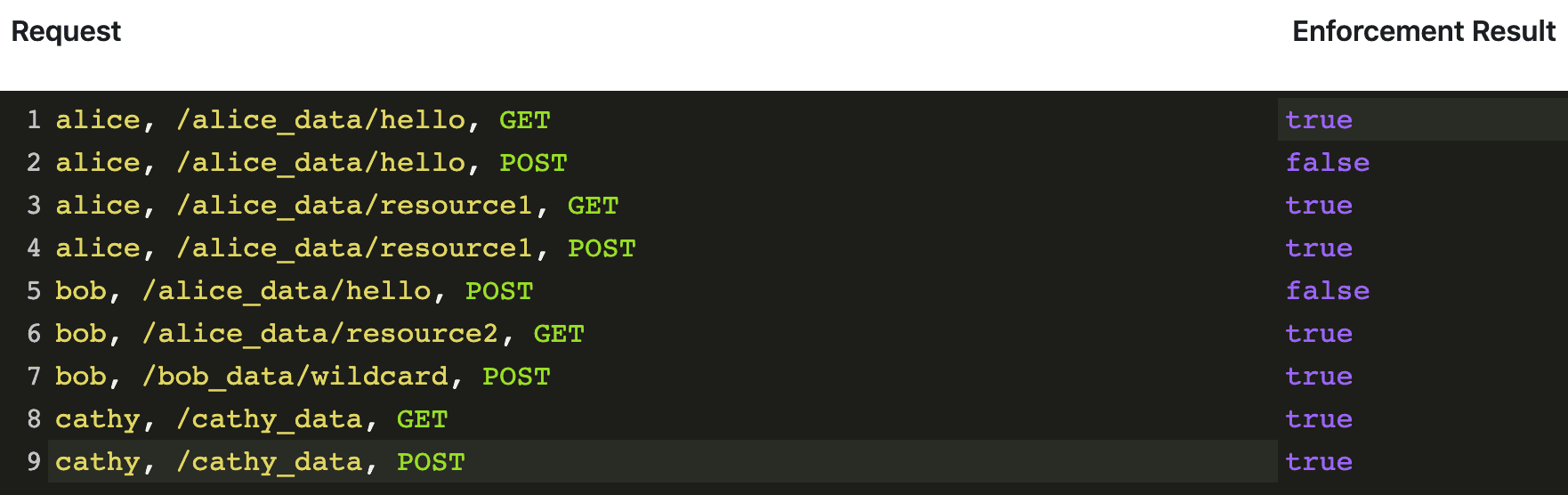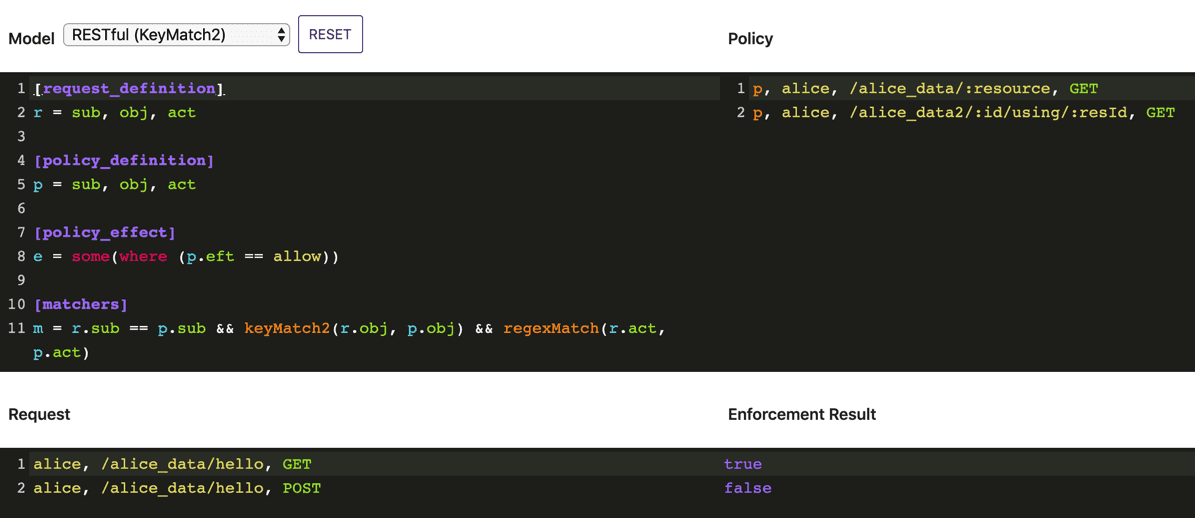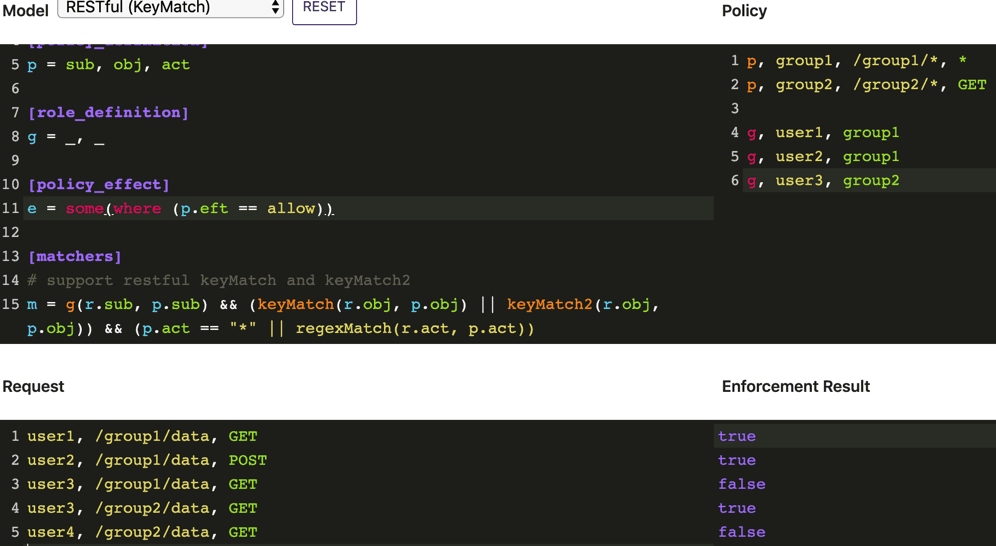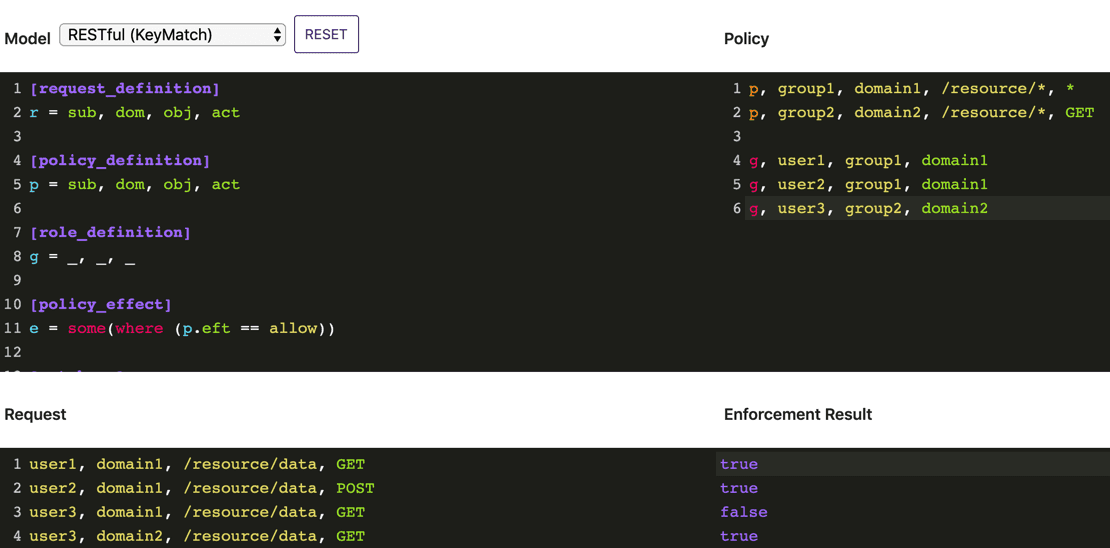## Casbin 是什么？⌗

`Casbin` 是一个强大的、高效的开源访问控制框架，其权限管理机制支持多种访问控制模型。它的强大之处在于:

• 支持多种语言(可在官网查看)
• 支持多种模型(可在官网查看)
• 策略持久化支持各种适配器(可在官网查看)

## 简单使用⌗

``````import { newEnforcer } from 'casbin'
import { authz, Authorizer } from '@zcong/koa-casbin'

// 鉴权中间件，为了简单，通过 header 中 user 字段来区分用户
// 未登录用户当做默认用户
function authMiddleware(): Koa.Middleware {
return async (ctx, next) => {
await next()
}
}
class MyAuthorizer extends Authorizer {
// 覆盖默认方法，取到鉴权中间件中的用户
return ctx.user
}
}
// 先使用鉴权中间件
app.use(authMiddleware())
// 使用 casbin 中间件
app.use(
authz({
newEnforcer: async () => {
const enforcer = await newEnforcer(
`\${__dirname}/authz_model.conf`, // 载入模型
`\${__dirname}/authz_policy.csv` // 载入策略
)
return enforcer
},
authorizer: MyAuthorizer,
})
)
``````

``````# authz_model.conf
[request_definition]
r = sub, obj, act

[policy_definition]
p = sub, obj, act

[policy_effect]
e = some(where (p.eft == allow))

[matchers]
m = r.sub == p.sub && keyMatch(r.obj, p.obj) && regexMatch(r.act, p.act)
``````

``````# authz_policy.csv
p, alice, /alice_data/*, GET
p, alice, /alice_data/resource1, POST

p, bob, /alice_data/resource2, GET
p, bob, /bob_data/*, POST

p, cathy, /cathy_data, (GET)|(POST)
``````## 更多模型⌗

### 另一种 Restful⌗``````[request_definition]
r = sub, obj, act

[policy_definition]
p = sub, obj, act

[role_definition]
g = _, _

[policy_effect]
e = some(where (p.eft == allow))

[matchers]
# support restful keyMatch and keyMatch2
m = g(r.sub, p.sub) && (keyMatch(r.obj, p.obj) || keyMatch2(r.obj, p.obj)) && (p.act == "*" || regexMatch(r.act, p.act))
``````

### Restful + RBAC⌗

``````[request_definition]
r = sub, obj, act

[policy_definition]
p = sub, obj, act

[role_definition]
g = _, _

[policy_effect]
e = some(where (p.eft == allow))

[matchers]
# support restful keyMatch and keyMatch2
m = g(r.sub, p.sub) && (keyMatch(r.obj, p.obj) || keyMatch2(r.obj, p.obj)) && (p.act == "*" || regexMatch(r.act, p.act))
``````### Restful + RBAC + domains⌗

``````[request_definition]
r = sub, dom, obj, act

[policy_definition]
p = sub, dom, obj, act

[role_definition]
g = _, _, _

[policy_effect]
e = some(where (p.eft == allow))

[matchers]
# support restful keyMatch and keyMatch2
m = g(r.sub, p.sub, r.dom) && r.dom == p.dom && (keyMatch(r.obj, p.obj) || keyMatch2(r.obj, p.obj)) && (p.act == "*" || regexMatch(r.act, p.act))
``````